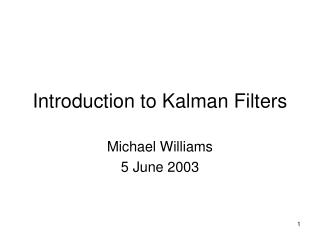# Introduction to Kalman Filters - PowerPoint PPT PresentationDownload PresentationIntroduction to Kalman Filters

Introduction to Kalman Filters
Download Presentation## Introduction to Kalman Filters

- - - - - - - - - - - - - - - - - - - - - - - - - - - E N D - - - - - - - - - - - - - - - - - - - - - - - - - - -
##### Presentation Transcript

1. Introduction to Kalman Filters Michael Williams 5 June 2003

2. Overview • The Problem – Why do we need Kalman Filters? • What is a Kalman Filter? • Conceptual Overview • The Theory of Kalman Filter • Simple Example

3. The Problem Black Box System Error Sources • System state cannot be measured directly • Need to estimate “optimally” from measurements External Controls System System State (desired but not known) Optimal Estimate of System State Observed Measurements Measuring Devices Estimator Measurement Error Sources

4. What is a Kalman Filter? • Recursive data processing algorithm • Generates optimal estimate of desired quantities given the set of measurements • Optimal? • For linear system and white Gaussian errors, Kalman filter is “best” estimate based on all previous measurements • For non-linear system optimality is ‘qualified’ • Recursive? • Doesn’t need to store all previous measurements and reprocess all data each time step

5. Conceptual Overview • Simple example to motivate the workings of the Kalman Filter • Theoretical Justification to come later – for now just focus on the concept • Important: Prediction and Correction

6. Conceptual Overview • Lost on the 1-dimensional line • Position – y(t) • Assume Gaussian distributed measurements y

7. Conceptual Overview • Sextant Measurement at t1: Mean = z1 and Variance = z1 • Optimal estimate of position is: ŷ(t1) = z1 • Variance of error in estimate: 2x(t1) = 2z1 • Boat in same position at time t2 - Predicted position is z1

8. Conceptual Overview prediction ŷ-(t2) measurement z(t2) • So we have the prediction ŷ-(t2) • GPS Measurement at t2: Mean = z2 and Variance = z2 • Need to correct the prediction due to measurement to get ŷ(t2) • Closer to more trusted measurement – linear interpolation?

9. Conceptual Overview prediction ŷ-(t2) corrected optimal estimate ŷ(t2) measurement z(t2) • Corrected mean is the new optimal estimate of position • New variance is smaller than either of the previous two variances

10. Conceptual Overview • Lessons so far: Make prediction based on previous data - ŷ-, - Take measurement – zk, z Optimal estimate (ŷ) = Prediction + (Kalman Gain) * (Measurement - Prediction) Variance of estimate = Variance of prediction * (1 – Kalman Gain)

11. Conceptual Overview ŷ(t2) Naïve Prediction ŷ-(t3) • At time t3, boat moves with velocity dy/dt=u • Naïve approach: Shift probability to the right to predict • This would work if we knew the velocity exactly (perfect model)

12. Conceptual Overview Naïve Prediction ŷ-(t3) ŷ(t2) Prediction ŷ-(t3) • Better to assume imperfect model by adding Gaussian noise • dy/dt = u + w • Distribution for prediction moves and spreads out

13. Conceptual Overview Corrected optimal estimate ŷ(t3) Measurement z(t3) Prediction ŷ-(t3) • Now we take a measurement at t3 • Need to once again correct the prediction • Same as before

14. Conceptual Overview • Lessons learnt from conceptual overview: • Initial conditions (ŷk-1 and k-1) • Prediction (ŷ-k , -k) • Use initial conditions and model (eg. constant velocity) to make prediction • Measurement (zk) • Take measurement • Correction (ŷk , k) • Use measurement to correct prediction by ‘blending’ prediction and residual – always a case of merging only two Gaussians • Optimal estimate with smaller variance

15. Theoretical Basis • Process to be estimated: yk = Ayk-1 + Buk + wk-1 Process Noise (w) with covariance Q zk = Hyk + vk Measurement Noise (v) with covariance R • Kalman Filter Predicted: ŷ-k is estimate based on measurements at previous time-steps ŷ-k = Ayk-1 + Buk P-k = APk-1AT + Q Corrected: ŷk has additional information – the measurement at time k ŷk = ŷ-k + K(zk - H ŷ-k ) K = P-kHT(HP-kHT + R)-1 Pk = (I - KH)P-k

16. Blending Factor • If we are sure about measurements: • Measurement error covariance (R) decreases to zero • K decreases and weights residual more heavily than prediction • If we are sure about prediction • Prediction error covariance P-k decreases to zero • K increases and weights prediction more heavily than residual

17. Correction (Measurement Update) Prediction (Time Update) (1) Compute the Kalman Gain (1) Project the state ahead K = P-kHT(HP-kHT + R)-1 ŷ-k = Ayk-1 + Buk (2) Update estimate with measurement zk (2) Project the error covariance ahead ŷk = ŷ-k + K(zk - H ŷ-k ) P-k = APk-1AT + Q (3) Update Error Covariance Pk = (I - KH)P-k Theoretical Basis

18. Quick Example – Constant Model Black Box System Error Sources External Controls System System State Optimal Estimate of System State Observed Measurements Measuring Devices Estimator Measurement Error Sources

19. Quick Example – Constant Model Prediction ŷ-k = yk-1 P-k = Pk-1 Correction K = P-k(P-k + R)-1 ŷk = ŷ-k + K(zk - H ŷ-k ) Pk = (I - K)P-k

20. Quick Example – Constant Model

21. Quick Example – Constant Model Convergence of Error Covariance - Pk

22. Quick Example – Constant Model Larger value of R – the measurement error covariance (indicates poorer quality of measurements) Filter slower to ‘believe’ measurements – slower convergence

23. References • Kalman, R. E. 1960. “A New Approach to Linear Filtering and Prediction Problems”, Transaction of the ASME--Journal of Basic Engineering, pp. 35-45 (March 1960). • Maybeck, P. S. 1979. “Stochastic Models, Estimation, and Control, Volume 1”, Academic Press, Inc. • Welch, G and Bishop, G. 2001. “An introduction to the Kalman Filter”, http://www.cs.unc.edu/~welch/kalman/